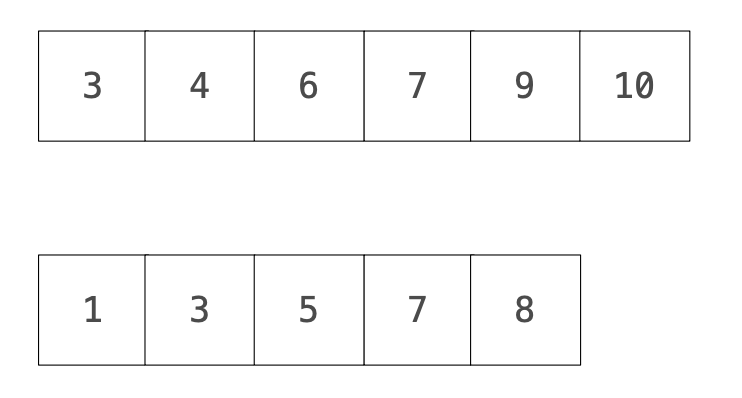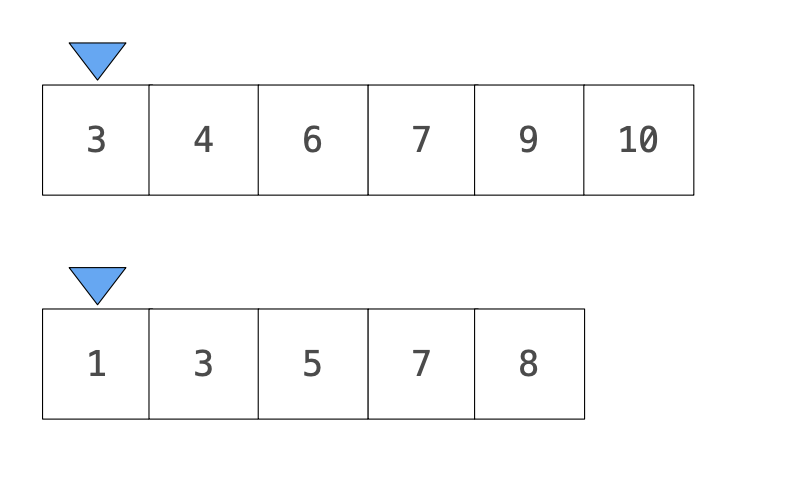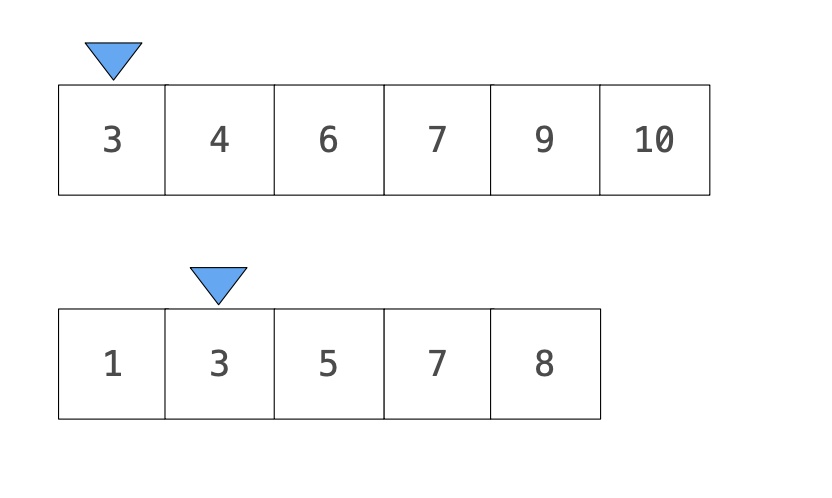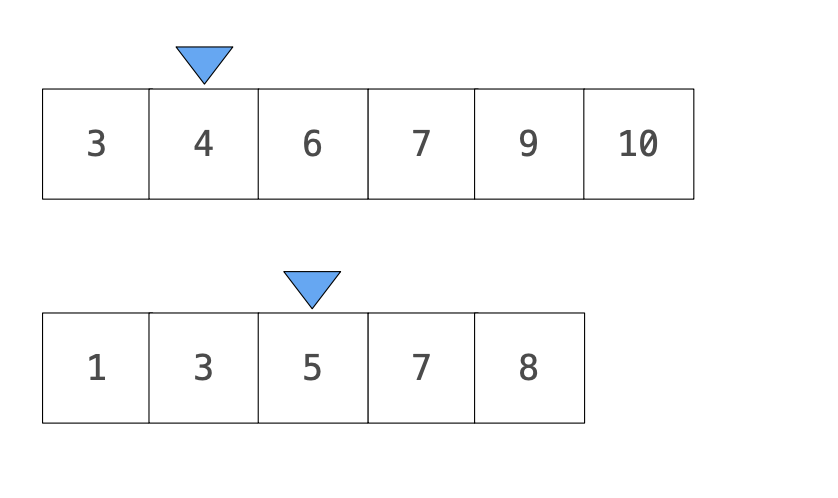# Python -「快速」算出兩排序數列中重複的次數

def overlap(s,t):
"""Return the overlap between S and T."""
>> overlap([3, 5, 4, 6, 9, 7, 10],[7, 3, 5, 1, 8])
>> 3
count = 0
for i in s:
for j in t:
if i == j:
count+=1
return count


def fast_overlap(s, t):
"""Return the overlap between sorted S and sorted T.
>> fast_overlap([3, 4, 5, 6, 7, 9, 10],[1, 3, 5, 7, 8])
>>3
"""
i, j,count = 0,0,0
while i < len(s) and j < len(t):
if s[i]==t[j]:
count, i, j = count+1, i+1, j+1
elif s[i] < t[j]:
i += 1
else:
j += 1
return count